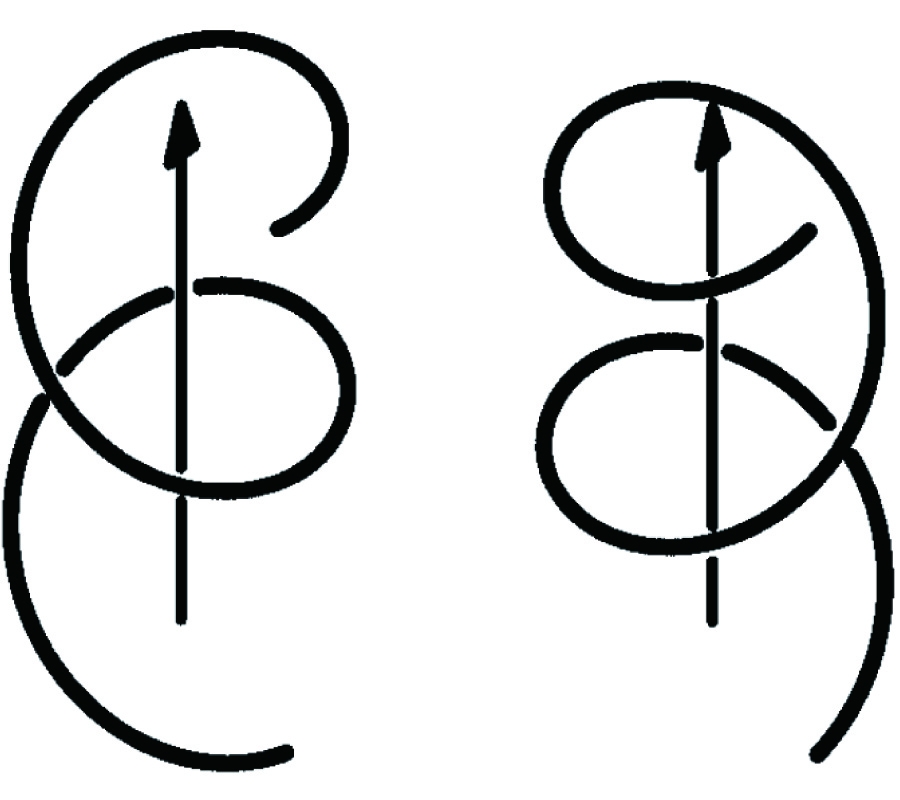# Vol. 46

Front:[PDF file] Back:[PDF file]
Latest Volume
All Volumes
All Issues
2004-06-08

#### Contact Geometry in Electromagnetism

By Matias Dahl
Progress In Electromagnetics Research, Vol. 46, 77-104, 2004
doi:10.2528/PIER03070801

## Abstract

In the first part of this work we show that, by working in Fourier space, the Bohren decomposition and the Helmholtz's decomposition can be combined into one decomposition. This yields a completely mathematical decomposition, which decomposes an arbitrary vector field on â„œ3 into three components. A key property of the decomposition is that it commutes both with the curl operator and with the time derivative. We can therefore apply this decomposition to Maxwell's equations without assuming anything about the media. As a result, we show that Maxwell's equations split into three completely uncoupled sets of equations. Further, when a medium is introduced, these decomposed Maxwell's equations either remain uncoupled, or become coupled depending on the complexity of the medium. In the second part of this work, we give a short introduction to contact geometry and then study its relation to electromagnetism. By studying examples, we show that the decomposed fields in the decomposed Maxwell's equations always seem to induce contact structures. For instance, for a plane wave, the decomposed fields are the right and left hand circulary polarized components, and each of these induce their own contact structure. Moreover, we show that each contact structure induces its own Carnot-CarathÃ©odory metric, and the path traversed by the circulary polarized waves seem to coincide with the geodesics of these metrics. This article is an abridged version of the author's master's thesis written under the instruction of Doctor Kirsi Peltonen and under the supervision of Professor Erkki Somersalo.

## Citation

Matias Dahl, "Contact Geometry in Electromagnetism," Progress In Electromagnetics Research, Vol. 46, 77-104, 2004.
doi:10.2528/PIER03070801
http://www.jpier.org/PIER/pier.php?paper=0307081

## References

1. Lindell, I. V., Methods for Electromagnetic Field Analysis, Clarendon Press, 1992.

2. Lindell, I. V., A. H. Sihvola, S. A. Tretyakov, and A. J. Viitanen, Electromagnetic Waves in Chiral and Bi-Isotropic Media, Artech House, 1994.

3. Hegstrom, R. A. and D. K. Kondepudi, "The handedness of the universe," Scientific American, No. 1, 98-105, 1990.

4. Gardner, M., The Ambidextrous Universe, Allen Lane, The Penguin Press, 1967.

5. Hargittai, I. and C. A. Pickover (eds.), Spiral Symmetry, World Scientific, 1992.

6. Moses, H. E., "Eigenfunctions of the curl operator; rotationally invariant Helmholtz theorem, and applications to electromagnetic theory and fluid mechanics," SIAM Journal of Applied Mathematics, Vol. 21, No. 1, 1971.
doi:10.1137/0121015

7. Waleffe, F., "The nature of triad interactions in homogeneous turbulence," Physics of Fluids A, Vol. 4, No. 2, 1992.

8. MacLeod, M. A., "The spherical curl transform of a linear forcefree magnetic field," Journal of Mathematical Physics, Vol. 39, No. 3, 1998.
doi:10.1063/1.532305

9. MacLeod, M. A., "A new description of force-free magnetic field," Journal of Mathematical Physics, Vol. 36, No. 6, 1995.
doi:10.1063/1.531003

10. Constantin, P. and A. Majda, "The Beltrami spectrum for incompressible fluid flows," Communications in Mathematical Physics, Vol. 115, 435-456, 1998.
doi:10.1007/BF01218019

11. Dahl, M., "Contact and symplectic geometry in electromagnetism," Master's thesis, 2002.

12. Arnold, V. I. and B. A. Keshin, "Topological methods in hydrodynamics," Applied Mathematical Sciences, 1998.

13. Baldwin, P. R. and G. M. Townsend, "Complex Trkalian fields and solutions to Euler's equations for the ideal fluid," Physical Review E, Vol. 51, No. 3, 2059-2068, 1995.
doi:10.1103/PhysRevE.51.2059

14. Tsui, K. H., "Force-free field model of ball lightning," Physics of Plasmas, Vol. 8, No. 3, 687-689, 2001.
doi:10.1063/1.1343511

15. Bellan, P. M., Spheromaks, A practical Application ofMagnetohydrodynamic Dynamos and Plasma Self-Organization, Imperial College Press, 2000.

16. Reed, D., Foundational Electrodynamics and Beltrami fields in in Advanced Electromagnetism: Foundations, Theory, and Applications, World Scientific, 1995.

17. Lakhtakia, A., Beltrami fields in Chiral Media, World Scientific, 1994.

18. Etnyre, J. and R. Ghrist, "Contact topology and hydrodynamics.," Nonlinearity, Vol. 13, No. 2, 441-458, 2000.
doi:10.1088/0951-7715/13/2/306

19. Stein, E. M. and G. Weiss, Introduction to Fourier Analysis on Euclidian Spaces, Princeton University Press, 1971.

20. Van Bladel, J., "A discussion on Helmholtz' theorem," Electromagnetics, Vol. 13, 95-110, 1993.

21. McDuff, D. and D. Salamon, Introduction to Symplectic Topology, Clarendon Press, 1997.

22. Madsen, I. and J. Tornehave, From Calculus to Cohomology, Cambridge University Press, 1997.

23. Boothby, W. M., An Introduction to Differentiable Manifolds and Riemannian Geometry, Academic Press, 1986.

24. Frankel, T., Geometry ofPhysics, Cambridge University Press, 1997.

25. Thirring, W., A Course in Mathematical Physics 2: Classical Field Theory, Springer-Verlag, 1978.
doi:10.1016/S0723-0869(01)80014-1

26. Geiges, H., "A brief history of contact geometry and topology," Expositiones Mathematicae, Vol. 19, 25-53, 2001.
doi:10.1016/S0723-0869(01)80014-1

27. BellaÃ¯che, A. and J-J. Risler (eds.), Sub-Riemannian Geometry, BirkhÃ¤user, 1996.
doi:10.1016/S0926-2245(02)00059-1

28. Beltran, J. V., "Star calculus on Jacobi manifolds," Differential Geometry and Its Applications, Vol. 16, 181-198, 2002.
doi:10.1016/S0926-2245(02)00059-1